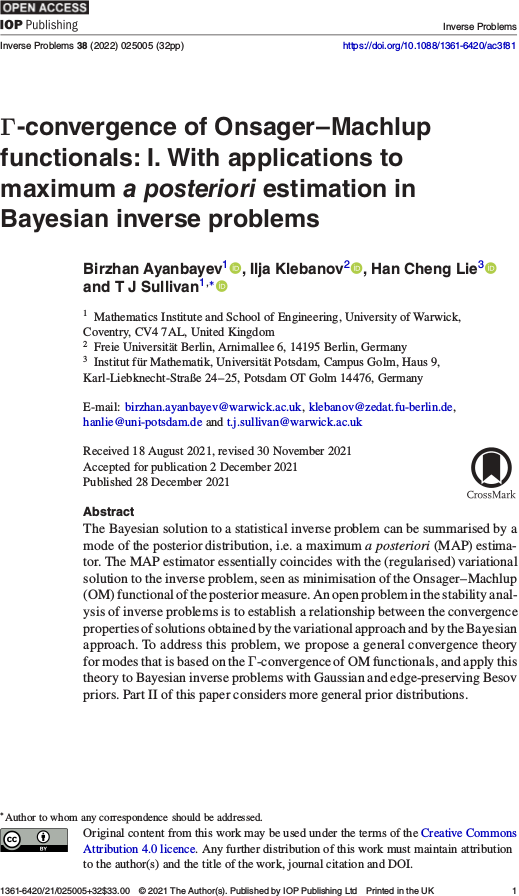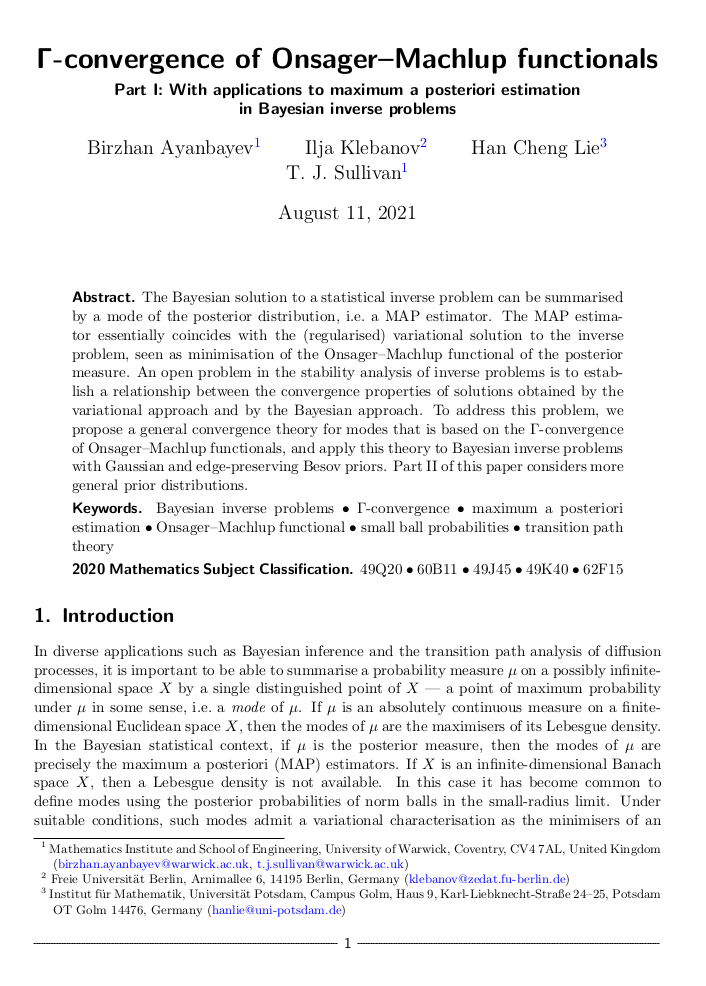# Tim Sullivan

### #modes

Clear Search### Order-theoretic perspectives on MAP estimation in SIAM/ASA JUQ

The final version of “An order-theoretic perspective on modes and maximum a posteriori estimation in Bayesian inverse problems” by Hefin Lambley and myself has just appeared online in the SIAM/ASA Journal on Uncertainty Quantification.

On a heuristic level, modes and MAP estimators are intended to be the “most probable points” of a space $$X$$ with respect to a probability measure $$\mu$$. Thus, in some sense, they would seem to be the greatest elements of some order on $$X$$, and a rigorous order-theoretic treatment is called for, especially for cases in which $$X$$ is, say, an infinite-dimensional function space. Such an order-theoretic perspective opens up some attractive proof strategies for the existence of modes and MAP estimators but also leads to some interesting counterexamples. In particular, because the orders involved are not total, some pairs of points of $$X$$ can be incomparable (i.e. neither is more nor less likely than the other). In fact we show that there are examples for which the collection of such mutually incomparable elements is dense in $$X$$.

H. Lambley and T. J. Sullivan. “An order-theoretic perspective on modes and maximum a posteriori estimation in Bayesian inverse problems.” SIAM/ASA Journal on Uncertainty Quantification 11(4):1195–1224, 2023. doi:10.1137/22M154243X

More →

Published on Friday 20 October 2023 at 09:00 UTC #publication #modes #order-theory #map-estimators #lambley #juq### A periodic table of modes and MAP estimators

Ilja Klebanov and I have just uploaded a preprint of our paper “A ‘periodic table’ of modes and maximum a posteriori estimators” to the arXiv.

This paper forms part of the growing body of work on the ‘small balls’ theory of modes for probability measures on metric spaces, which is needed e.g. for the treatment of MAP estimation for Bayesian inverse problems with functional unknowns. There are already several versions in the literature: the strong mode, the weak mode, and the generalised strong mode. We take an axiomatic approach to the problem and identify a system of 17 essentially distinct notions of mode, proving implications between them and providing explicit counterexamples to distinguish them. From an axiomatic point of view, all these 17 seem to be ‘equally good’, suggesting that further research is needed in this area.

Abstract. The last decade has seen many attempts to generalise the definition of modes, or MAP estimators, of a probability distribution $$\mu$$ on a space $$X$$ to the case that $$\mu$$ has no continuous Lebesgue density, and in particular to infinite-dimensional Banach and Hilbert spaces $$X$$. This paper examines the properties of and connections among these definitions. We construct a systematic taxonomy – or ‘periodic table’ – of modes that includes the established notions as well as large hitherto-unexplored classes. We establish implications between these definitions and provide counterexamples to distinguish them. We also distinguish those definitions that are merely ‘grammatically correct’ from those that are ‘meaningful’ in the sense of satisfying certain ‘common-sense’ axioms for a mode, among them the correct handling of discrete measures and those with continuous Lebesgue densities. However, despite there being 17 such ‘meaningful’ definitions of mode, we show that none of them satisfy the ‘merging property’, under which the modes of $$\mu|_{A}$$, $$\mu|_{B}$$, and $$\mu|_{A \cup B}$$ enjoy a straightforward relationship for well-separated positive-mass events $$A, B \subseteq X$$.

Published on Monday 17 July 2023 at 09:00 UTC #preprint #modes #map-estimators #klebanov### Order theory and MAP estimation

Hefin Lambley and I have just uploaded a preprint of our latest article, “An order-theoretic perspective on modes and maximum a posteriori estimation in Bayesian inverse problems”, to the arXiv. On a heuristic level, modes and MAP estimators are intended to be the “most probable points” of a space $$X$$ with respect to a probability measure $$\mu$$. Thus, in some sense, they would seem to be the greatest elements of some order on $$X$$, and a rigorous order-theoretic treatment is called for, especially for cases in which $$X$$ is, say, an infinite-dimensional function space. Such an order-theoretic perspective opens up some attractive proof strategies for the existence of modes and MAP estimators but also leads to some interesting counterexamples. In particular, because the orders involved are not total, some pairs of points of $$X$$ can be incomparable (i.e. neither is more nor less likely than the other). In fact we show that there are examples for which the collection of such mutually incomparable elements is dense in $$X$$.

Abstract. It is often desirable to summarise a probability measure on a space $$X$$ in terms of a mode, or MAP estimator, i.e. a point of maximum probability. Such points can be rigorously defined using masses of metric balls in the small-radius limit. However, the theory is not entirely straightforward: the literature contains multiple notions of mode and various examples of pathological measures that have no mode in any sense. Since the masses of balls induce natural orderings on the points of $$X$$, this article aims to shed light on some of the problems in non-parametric MAP estimation by taking an order-theoretic perspective, which appears to be a new one in the inverse problems community. This point of view opens up attractive proof strategies based upon the Cantor and Kuratowski intersection theorems; it also reveals that many of the pathologies arise from the distinction between greatest and maximal elements of an order, and from the existence of incomparable elements of $$X$$, which we show can be dense in $$X$$, even for an absolutely continuous measure on $$X = \mathbb{R}$$.

Published on Monday 26 September 2022 at 09:00 UTC #preprint #modes #order-theory #map-estimators #lambley### Γ-convergence of Onsager-Machlup functionals in Inverse Problems

The articles “Γ-convergence of Onsager–Machlup functionals” (“I. With applications to maximum a posteriori estimation in Bayesian inverse problems” and “II. Infinite product measures on Banach spaces”) by Birzhan Ayanbayev, Ilja Klebanov, Han Cheng Lie, and myself have just appeared in their final form in the journal Inverse Problems.

The purpose of this work is to address a long-standing issue in the Bayesian approach to inverse problems, namely the joint stability of a Bayesian posterior and its modes (MAP estimators) when the prior, likelihood, and data are perturbed or approximated. We show that the correct way to approach this problem is to interpret MAP estimators as global weak modes in the sense of Helin and Burger (2015), which can be identified as the global minimisers of the Onsager–Machlup functional of the posterior distribution, and hence to provide a convergence theory for MAP estimators in terms of Γ-convergence of these Onsager–Machlup functionals. It turns out that posterior Γ-convergence can be assessed in a relatively straightforward manner in terms of prior Γ-convergence and continuous convergence of potentials (negative log-likelihoods). Over the two parts of the paper, we carry out this programme both in generality and for specific priors that are commonly used in Bayesian inverse problems, namely Gaussian and Besov priors (Lassas et al., 2009; Dashti et al., 2012).

B. Ayanbayev, I. Klebanov, H. C. Lie, and T. J. Sullivan. “Γ-convergence of Onsager–Machlup functionals: I. With applications to maximum a posteriori estimation in Bayesian inverse problems.” Inverse Problems 38(2):025005, 32pp., 2022. doi:10.1088/1361-6420/ac3f81

Abstract (Part I). The Bayesian solution to a statistical inverse problem can be summarised by a mode of the posterior distribution, i.e. a MAP estimator. The MAP estimator essentially coincides with the (regularised) variational solution to the inverse problem, seen as minimisation of the Onsager–Machlup functional of the posterior measure. An open problem in the stability analysis of inverse problems is to establish a relationship between the convergence properties of solutions obtained by the variational approach and by the Bayesian approach. To address this problem, we propose a general convergence theory for modes that is based on the Γ-convergence of Onsager–Machlup functionals, and apply this theory to Bayesian inverse problems with Gaussian and edge-preserving Besov priors. Part II of this paper considers more general prior distributions.

B. Ayanbayev, I. Klebanov, H. C. Lie, and T. J. Sullivan. “Γ-convergence of Onsager–Machlup functionals: II. Infinite product measures on Banach spaces.” Inverse Problems 38(2):025006, 35pp., 2022. doi:10.1088/1361-6420/ac3f82

Abstract (Part II). We derive Onsager–Machlup functionals for countable product measures on weighted $$\ell^{p}$$ subspaces of the sequence space $$\mathbb{R}^\mathbb{N}$$. Each measure in the product is a shifted and scaled copy of a reference probability measure on $$\mathbb{R}$$ that admits a sufficiently regular Lebesgue density. We study the equicoercivity and Γ-convergence of sequences of Onsager–Machlup functionals associated to convergent sequences of measures within this class. We use these results to establish analogous results for probability measures on separable Banach or Hilbert spaces, including Gaussian, Cauchy, and Besov measures with summability parameter $$1 \leq p \leq 2$$. Together with Part I of this paper, this provides a basis for analysis of the convergence of maximum a posteriori estimators in Bayesian inverse problems and most likely paths in transition path theory.

Published on Wednesday 5 January 2022 at 12:00 UTC #publication #inverse-problems #modes #map-estimators #ayanbayev #klebanov #lie### Γ-convergence of Onsager-Machlup functionals

Birzhan Ayanbayev, Ilja Klebanov, Han Cheng Lie, and I have just uploaded preprints of our work “Γ-convergence of Onsager–Machlup functionals” to the arXiv; this work consists of two parts, “Part I: With applications to maximum a posteriori estimation in Bayesian inverse problems” and “Part II: Infinite product measures on Banach spaces”.

The purpose of this work is to address a long-standing issue in the Bayesian approach to inverse problems, namely the joint stability of a Bayesian posterior and its modes (MAP estimators) when the prior, likelihood, and data are perturbed or approximated. We show that the correct way to approach this problem is to interpret MAP estimators as global weak modes in the sense of Helin and Burger (2015), which can be identified as the global minimisers of the Onsager–Machlup functional of the posterior distribution, and hence to provide a convergence theory for MAP estimators in terms of Γ-convergence of these Onsager–Machlup functionals. It turns out that posterior Γ-convergence can be assessed in a relatively straightforward manner in terms of prior Γ-convergence and continuous convergence of potentials (negative log-likelihoods). Over the two parts of the paper, we carry out this programme both in generality and for specific priors that are commonly used in Bayesian inverse problems, namely Gaussian and Besov priors (Lassas et al., 2009; Dashti et al., 2012).

Abstract (Part I). The Bayesian solution to a statistical inverse problem can be summarised by a mode of the posterior distribution, i.e. a MAP estimator. The MAP estimator essentially coincides with the (regularised) variational solution to the inverse problem, seen as minimisation of the Onsager–Machlup functional of the posterior measure. An open problem in the stability analysis of inverse problems is to establish a relationship between the convergence properties of solutions obtained by the variational approach and by the Bayesian approach. To address this problem, we propose a general convergence theory for modes that is based on the Γ-convergence of Onsager–Machlup functionals, and apply this theory to Bayesian inverse problems with Gaussian and edge-preserving Besov priors. Part II of this paper considers more general prior distributions.

Abstract (Part II). We derive Onsager–Machlup functionals for countable product measures on weighted $$\ell^{p}$$ subspaces of the sequence space $$\mathbb{R}^\mathbb{N}$$. Each measure in the product is a shifted and scaled copy of a reference probability measure on $$\mathbb{R}$$ that admits a sufficiently regular Lebesgue density. We study the equicoercivity and Γ-convergence of sequences of Onsager–Machlup functionals associated to convergent sequences of measures within this class. We use these results to establish analogous results for probability measures on separable Banach or Hilbert spaces, including Gaussian, Cauchy, and Besov measures with summability parameter $$1 \leq p \leq 2$$. Together with Part I of this paper, this provides a basis for analysis of the convergence of maximum a posteriori estimators in Bayesian inverse problems and most likely paths in transition path theory.

Published on Wednesday 11 August 2021 at 12:00 UTC #preprint #inverse-problems #modes #map-estimators #ayanbayev #klebanov #lie